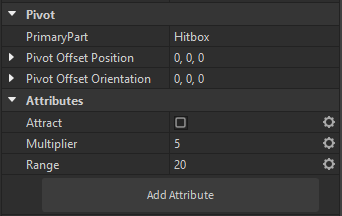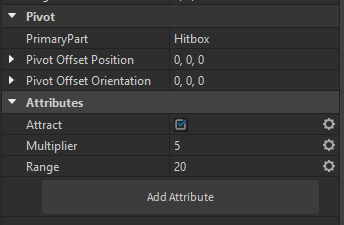# Magnet script only works on a single instance of the object. (kinda)

I am making a Magnet script that either attracts or pushes away the player.

But the script, for some reason, only does one part of the script. From the location of the first found Instance.

Example:

Here is the script that handles the magnets:

``````-- Magnet Logic
rs:BindToRenderStep("magnetize",422,function(t)
for i, mag in pairs(workspace.Magnetize_:GetChildren()) do
--print(v,v.PrimaryPart.Position,pushVector,npushVector)
local r = mag:GetAttribute("Range")
local m = mag:GetAttribute("Multiplier")
print(mag:GetAttribute("Attract"))
if mag:GetAttribute("Attract") == false then
if mag.PrimaryPart.Position.Magnitude - plrb.Position.Magnitude <= r then
local pushVector = plrb.Position - mag.PrimaryPart.Position
--print(mag,"Repulsor")
plrb.AssemblyLinearVelocity = plrb.AssemblyLinearVelocity + pushVector*m
end
elseif mag:GetAttribute("Attract") == true then
if mag.PrimaryPart.Position.Magnitude - plrb.Position.Magnitude <= r then
local npushVector = mag.PrimaryPart.Position - plrb.Position
--print(mag,"Attractor")
plrb.AssemblyLinearVelocity = plrb.AssemblyLinearVelocity + npushVector*m
end
end
end
end)
``````

plrb is the player’s ball

Here is the configuration im using for the parts.andFrom many prints, I know that mag is going through every instance like its supposed to and displays the correct Attract boolean, but still triggers the first instance when I enter the area of the 2nd instance.

``````if mag.PrimaryPart.Position.Magnitude - plrb.Position.Magnitude <= r then
``````

This equation does not return a distance check. It’s close to this which is likely what you want, an actual distance check.

``````if (mag.PrimaryPart.Position - plrb.Position).Magnitude <= r then
``````
1 Like